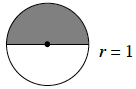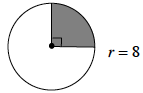### Home > MC2 > Chapter 6 > Lesson 6.1.4 > Problem6-41

6-41.

Find the area of the shaded portion of each circle.

1.Remember the area of a circle is $r^2π$.

To find the area of the entire circle, substitute $1$ for $r$: Area $=(1^2)π$

Since only half the circle is shaded, we only need half of the entire area calculated. Therefore, divide your answer by $2$.

It is about $1.57$ square units.

1.See part (a). This time only one-fourth of the circle is shaded.